# Logistic Growth and SIR Modelling of Coronavirus Disease (COVID-19) Outbreak in India: Models Based on Real-Time Data

Logistic Growth and SIR Modelling of Coronavirus Disease (COVID-19) Outbreak in India: Models Based on Real-Time Data
Department of Mathematics, CHRIST (Deemed to be University), Bengaluru 560029, India

Corresponding Author Email:
mahanthesh.b@christuniversity.in
Page:
345-350
|
DOI:
https://doi.org/10.18280/mmep.070303
25 May 2020
|
Accepted:
7 July 2020
|
Published:
30 September 2020
| Citation

OPEN ACCESS

Abstract:

The logistic growth model and the Susceptible-Infectious-Recovered (SIR) framework are utilized for the mathematical modelling of the Coronavirus disease (COVID-19) outbreak in India. Karnataka, Kerala and Maharashtra, three states of India, are selected based on the pattern of the disease spread and the prominence in being affected in India. The parameters of the models are estimated by utilizing real-time data. The models predict the ending of the pandemic in these states and estimate the number of people that would be affected under the prevailing conditions. The models classify the pandemic into five stages based on the nature of the infection growth rate. According to the estimates of the models it can be concluded that Kerala is in a stable situation whereas the pandemic is still growing in Karnataka and Maharashtra. The infection rate of Karnataka and Kerala are lesser than 5% and reveal a downward trend. On the other hand, the infection rate and the high predicted number of infectives in Maharashtra calls for more preventive measures to be imposed in Maharashtra to control the disease spread. The results of this analysis provide valuable information regarding the disease spread in India.

Keywords:

COVID-19, epidemic, logistic growth model, mathematical modelling, novel Corona virus, SIR model

1. Introduction

The novel Corona virus disease-2019 (COVID-19) is a deadly infectious disease which was first reported in Wuhan, China by the end of the year 2019. Later, it spread across continents leaving only a few countries unaffected. The large spread and increasing number of deaths made the World Health Organization (WHO) to declare it as a pandemic on 11 March 2020 . The governments of the affected countries have imposed various preventive measures including lockdown, seal down, social distancing, quarantining infected and susceptible individuals, promotion of self-hygiene measures and usage of masks. Recently, Fang et al.  reported that the governmental interventions proved to be effective in containing the infection rate.

In India, the pandemic was first reported on 30 January 2020 in Thrissur, a district of Kerala for three individuals who had come from Wuhan. In March, the number of infected individuals multiplied due to individuals who had travelled to India from various affected countries. Initially, the government imposed preventive measures including thermal screening of passengers arriving at the airport, shut down of educational institutions and suspending tourist visas. Further, on 24 March, the government imposed a nationwide lock down as a major preventive measure to contain the spread of the virus. This restriction on the human mobility was reported to be effective for various infectious diseases [3, 4]. The Indian context of the disease spread and the impact of the public health interventions were mathematically modelled by Mandal et al. .

During these hard times of the pandemic, researchers from various disciplines conduct intensive research to analyze it and to curb its ill-effects [6-8]. Apart from virologic and experimental studies, mathematical and statistical explorations are also important for the decision-makers and policy makers to evaluate the current situation and to take the necessary actions for the future. These models also provide an estimate of the ending time and possible number of people that can be infected by this pandemic. The logistic growth model is an efficient technique for epidemic forecasting. Chowell et al.  used this model to estimate the transmission of the Ebola virus. They concluded that the predictions from the model were inconsistent in the initial period of the epidemic. The model was also adopted by Pell et al.  to estimate the final size and the peak time of the infection. The SIR model (compartmental disease model) is a mathematical method to describe the epidemic growth through a system of time dependent differential equations. The differential equations are based on the compartments into which the population is divided. The SIR model and various modified SIR models were widely used by researchers to model Ebola  and AIDS . Recently, such models were used to model the coronavirus epidemic spreading. Khrapov and Loginova  utilized the modified SIR model for the analyzing the dynamics of the COVID-19 pandemic in China. In this direction, the modified SEIR model was used by Berger et al.  to include the effects of quarantining and infection testing.

Aggravated by the findings of the above explorations, the present article aims at studying the dynamics of the novel Corona virus (COVID-19) in India. The mathematical modelling of the COVID-19 outbreak is done for three states of India, namely Karnataka, Kerala and Maharashtra due to the significant difference in the outbreak pattern. The logistic growth model and the SIR model are used to analyze the situation in these states and the results of the models are compared. The simulations are carried out with the parameters that are estimated from the real time data by utilizing MATLAB. The data from 9 March 2020 to 9 May 2020 are used for the modelling. The final size of the pandemic in the states is estimated and the date when the pandemic becomes stable is also predicted.

2. Mathematical Models

2.1 Logistic growth model

The population growth can be modelled using the logistic growth model which is a sigmoid curve. Pierre-Francois Verhulst pioneered the usage of logistic growth models for biological systems.

$\frac{d \mathcal{C}}{d t}=r \mathcal{C}\left(1-\frac{\mathcal{C}}{K}\right)$  (1)

where,  C -accumulated number of cases,

r-infection rate,

t -time and

K is the final epidemic size.

If the initial number of cases is given by $\left.\mathcal{C}\right|_{t=0}=\mathcal{C}_{0}$, then the solution to Eq. (1) is

$\mathcal{C}=\frac{K \mathcal{C}_{0} e^{r t}}{K+\mathcal{C}_{0}\left(e^{r t}-1\right)}$  (2)

i.e., $\mathcal{C}=\frac{K}{1+A e^{-r t}}$ where $, A=\left(\frac{K-\mathcal{C}_{0}}{\mathcal{C}_{0}}\right)$.   (3)

For estimating the maximum number of affected people, the cases follow the Weibull function. Further, the highest growth rate occurs at the time $t_{\max }=\frac{\ln A}{r}$. At tmax, the number of cases is $\frac{K}{2}$. The epidemy is modelled using five stages based on the time tmax. This is based on the nature of the growth rate. The five stages are as follows:

Stage 1: Slow growth stage

The spread of any infectious disease is slow initially which is accounted in this stage. This is the exponential growth phase of the logistic growth model. The growth at the time $t<t_{\max }-\frac{2}{r}$ is considered in this stage.

Stage 2: Accelerating growth stage

Here the spread of the disease spreads largely in the population. At this stage the number of positive cases multiplies several folds. This accelerating phase is at the time $t_{\max }-\frac{2}{r}<t<t_{\max }$.

Stage 3: Decelerating growth stage

At this stage the spread of the disease decelerates due to various preventive measures taken. The spread of the disease is controlled and lesser than that of Stage 2. The decelerating growth is at the time $t_{m a x}<t<t_{m a x}+\frac{2}{r}$.

Stage 4: Transition stage

This is the stage when the disease growth rate slows down. At this stage the epidemy is said to be under control. The preventive measures taken prove to be effective. The time duration of this stage is $t_{\max }+\frac{2}{r}<t<2 t_{\max }$.

This stage can be regarded as the culminating stage of the epidemy. There is no growth in the disease spread. This stage is at the time t>2tmax.

2.2 SIR model

The Susceptible-Infected-Recovered (SIR) model is a mathematical model also known as compartmental disease model to describe the disease spread in a population. Here, the considered population belongs to any one of the three compartments: Susceptible, Infected or Recovered as shown in Figure 1.

## 1.jpg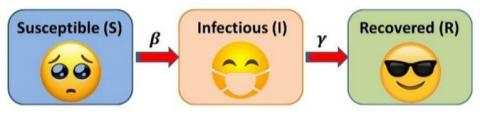Figure 1. SIR model

Susceptible are the individuals without immunity to the disease, hence they can be infected. Susceptible individuals can move to the next compartment (Infectious) through the interaction with infectious beings. Infectious beings are infected with the disease and they can transmit the disease to the susceptible individuals. By recovering from the illness, they can move to the next compartment called Recovered. Now, recovered beings can no longer develop the infection as they gained immunity via prior exposure. As beings can move among compartments, the number of members in each compartment varies with time. This model was first constructed by Kermack and McKendrick , it comprises of following set of time related nonlinear ordinary differential equations to simulate the growth of a disease.

$\frac{d S(t)}{d t}=-\frac{\beta}{N} S(t) I(t)$,  (4)

$\frac{d I(t)}{d t}=\frac{\beta}{N} S(t) I(t),-\gamma I(t)$,  (5)

$\frac{d R(t)}{d t}=\gamma I(t)$,  (6)

where, S(t) is the number of susceptible individuals,

I(t) is the number of infected individuals

R(t) is the number of recovered individuals

N is the considered constant population size involved in the disease

β is the contact rate of the disease

γ is the mean recovery/removal rate.

The initial conditions are S(t=0), I(t=0), R(t=0)≥0. Let it be respectively S0, I0 and R0.

The following suppositions are involved in the model:

•       The population is considered to be unvarying during the phase of modelling.

•       All the infected beings have an equal chance to be recovered.

•       Secondary waves of infections and any other unusual outbreak of the infection are not considered in these models.

•       The real time data of the officially reported positive cases are used for the models.

Solving the equations one can get,

$S(t)=S_{0} e^{-\frac{\beta}{N \gamma}\left(R(t)-R_{0}\right)}$  (7)

To find the total number of susceptible individuals at the end of the disease spread, the limit to $\infty$ is taken to get:

$S_{\infty}=S_{0} e^{-\frac{\beta}{N \gamma}\left(R_{\infty}-R_{0}\right)}$  (8)

where, Ris the final number of recovered individuals. In order to estimate the model parameters and S0 with the available real time data of the number of positive cases (denoted by Ci for each day i), the initial values are taken to be I0=C1 and R0=0. Now, the parameters are found such that the error sum of square of the predicted and the actual number of cases is minimum.

3. Methodology

The number of positive novel corona virus (COVID-19) cases in Karnataka, Kerala and Maharashtra from 9 March 2020 to 9 May 2020 were recorded. The data source was based on the daily reports from the respective health departments of the states. These states were selected due to the significant difference in the disease spread patterns. This data was used to estimate the parameters for the logistic growth model and the SIR model. The models and the visualizations are done using the fitvirus program [16, 17] using MATLAB.

4. Interpretations of the Results

4.1 Logistic growth model

Table 1 summarizes the estimated parameters for the logistic growth model. Within two months, Kerala reached the steady stage (Stage 5) whereas Maharashtra and Karnataka are in the decelerating growth stage (Stage 3). Though Karnataka and Maharashtra are in the same stage, the intensity of the disease spread is different. The logistic growth model for the three states is displayed in Figures 2-4.

Considering that the same situations prevail in the states and the present growth rates, the estimated maximum number of people who will be infected in Maharashtra, Karnataka and Kerala are 43993, 1009 and 485 respectively. It is to be noted that the prediction of Kerala is lesser underestimated. This can be due to secondary disease infection waves or migration. This is visualized in Figures 5-7. The duration of the accelerating growth stage and the date corresponding to tmax are tabulated in Table 1. The end of the transition stage can be regarded as the stage at which the disease spread is under control. It is interesting to note that the estimated end of the transition stage for Maharashtra is by 7 July while it is by 24 April in Kerala and 11 June in Karnataka. The high values of the coefficient of determination (R2) suggest the accuracy of the fitted models.

## 2.jpg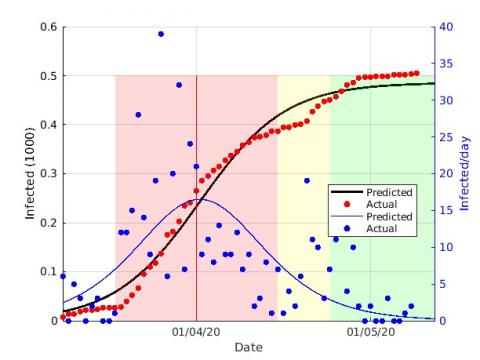Figure 2. Logistic growth modelling of COVID-19 cases in Kerala

## 3.jpgFigure 3. Logistic growth modelling of COVID-19 cases in Karnataka

## 4.jpg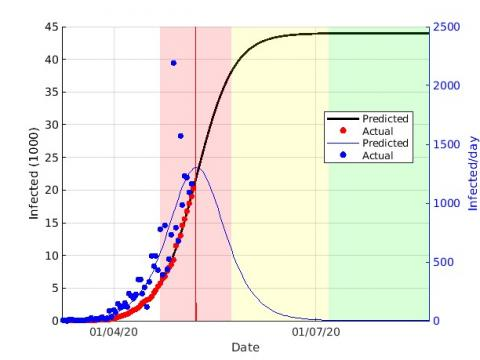Figure 4. Logistic growth modelling of COVID-19 cases in Maharashtra

Table 1. Estimated logistic growth model summary

 Parameter Kerala Karnataka Maharashtra Epidemic stage Stage 5 Stage 3 Stage 3 Number of cases on 9 May 2020 505 794 20228 Epidemic size (cases) 485 1009 43993 Duration of accelerating growth (days) 29 44 33 date of tmax 1-04-2020 25-04-2020 08-05-2020 End of transition stage 24-04-2020 11-06-2020 07-07-2020 Total R2 98.4% 99.5% 99.9%

## 5.jpg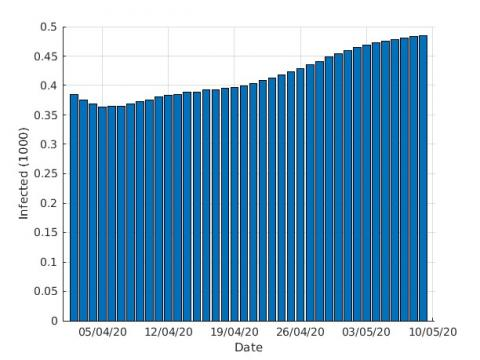Figure 5. Daily estimated final size of COVID-19 cases in Kerala

## 6.jpg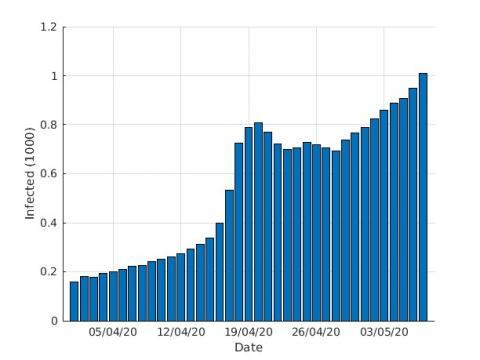Figure 6. Daily estimated final size of COVID-19 cases in Karnataka

## 7.jpg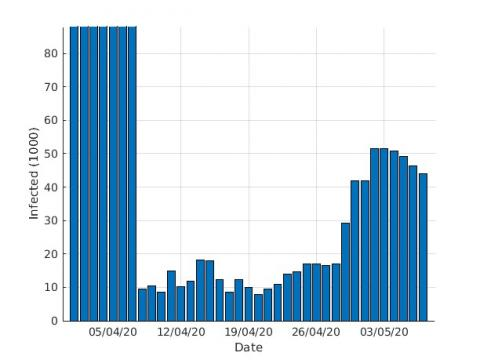Figure 7. Daily estimated final size of COVID-19 cases in Maharashtra

4.2 SIR model

The estimated parameters for the SIR model of the three states are given in Table 2. The contact rate quantifies the mean number of contacts per infected individual per day. It is seen that the contact rate is the highest for Maharashtra. The exclusion of the infected population by immunization, death, quarantining or isolation is quantified using the removal rate (γ). A high removal rate (γ=0.914) is estimated for Maharashtra. The estimated number of individuals who are likely to be involved in the coronavirus outbreak in each state is 487 (Kerala), 6626 (Karnataka) and 213604 (Maharashtra). In the aforementioned estimated numbers, the estimated number of infections in each state is found to be 449 (Kerala), 1177 (Karnataka) and 46678 (Maharashtra). The remaining individuals are the estimated number of susceptible individuals. Time between contacts is the reciprocal of the contact rate and the time between contacts is the reciprocal of the removal rate. The respective values of each state are recorded in Table 2. The basic reproduction number (R0) quantifies the expected number of secondary infections by one typical infection in a population that is completely susceptible. Based on the data it is estimated that the $R_{0}$ of Kerala is the highest among the three states. The high predicted number of cases of Maharashtra indicates that the state must enforce more strict measures to curb the spread of the virus.

The SIR models of the states are visualized in Figures 8-10. The estimated number of infection rate (using the SIR model) is also plotted against the actual number of cases/day. Further, the change in the number of cases is analysed by using the growth rate plot. If the growth rate of the number of new cases reported per day is lesser than 5%, then the outbreak is said to be under control. It can be concluded that the infection growth rate is lesser than 5% in Kerala and Karnataka but Maharashtra is yet to get control over the infection transmission.

## 8.jpg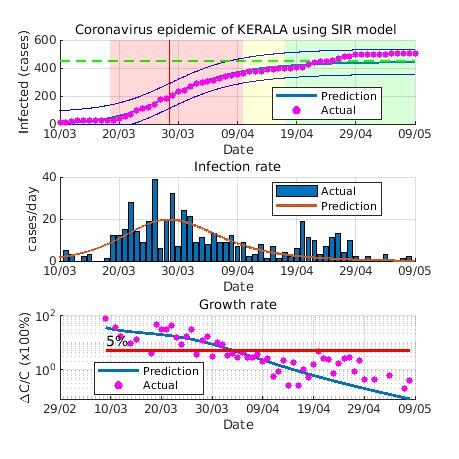Figure 8. SIR modelling of COVID-19 cases in Kerala

Table 2. Estimated susceptible-infected-recovered (SIR) model parameters

 Parameter Kerala Karnataka Maharashtra Contact rate ($\beta$) (/day) 0.312 0.836 1.031 Removal rate ($\gamma$) (/day) 0.114 0.76 0.914 Final number of cases 449 1177 46678 Final number of susceptible 38 5449 166926 Involved Population size (N) 487 6626 213604 Time between contacts (day) 3.2 1.2 1 Infectious period (day) 8.8 1.3 1.1 Basic reproduction number $\left(R_{0}\right)$ 2.73684 1.1 1.128

## 9.jpg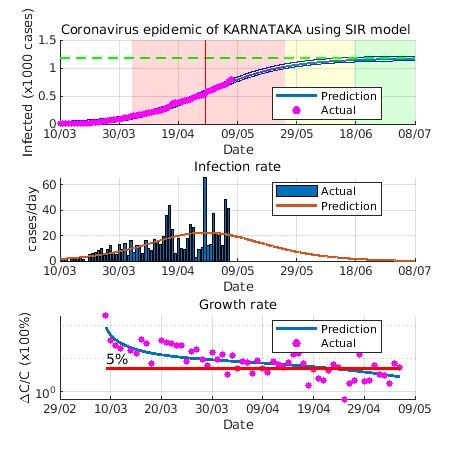Figure 9. SIR modelling of COVID-19 cases in Karnataka

## 10.jpgFigure 10. SIR modelling of COVID-19 cases in Maharashtra

5. Concluding Remarks

The COVID-19 outbreak in India was analyzed by comparing the dynamics of the pandemic in Karnataka, Kerala and Maharashtra using the logistic growth and SIR models. It was observed that the states experience a totally different pattern of the disease transmission. Maharashtra and Karnataka are found to be in the decelerating growth stage whereas Kerala is already in the steady stage. The strict and humane prevention measures taken by the government of Kerala may be the reason for the quick control over the pandemic. The size of the pandemic was estimated using the real time data of the daily reported cases. The logistic growth model predicted the maximum number infected individuals in Kerala, Karnataka and Maharashtra to be 485, 1009 and 43993 respectively whereas the SIR model estimated it to be 449, 1177 and 46678 respectively. The complete control of the disease spread is predicted to be by 7 July in Maharashtra while it is by 24 April in Kerala and 11 June in Karnataka. From the SIR model, it is concluded that the infection growth rate is lesser than 5% in Kerala and Karnataka but Maharashtra experiences is yet to achieve that state. The predicted values and dates are based on the prevalent conditions and pattern of disease spread for the considered time period. The high predicted number of infectives in Maharashtra calls for more preventive measures to be taken in the state.

Acknowledgment

The authors are thankful to the management of CHRIST (Deemed to be University) for their support.

Nomenclature
 COVID-19 Coronavirus disease 2019 SIR Susceptible-Infectious-Recovered WHO World Health Organization $\mathcal{C}$ accumulated number of cases r infection rate t time K final epidemic size S(t) number of susceptible individuals I(t) number of infected individuals R(t) number of recovered individuals N considered constant population size involved in the disease Greek symbols β contact rate of the disease γ mean recovery/removal rate Subscripts max maximum 0 initial number
References

 World Health Organization. “Coronavirus disease 2019”. Available: www.who.int/emergencies/diseases/novel-coronavirus-2019.

 Fang, Y., Nie, Y., Penny, M. (2020). Transmission dynamics of the COVID‐19 outbreak and effectiveness of government interventions: A data-driven analysis. Journal of Medical Virology, 92(6): 645-659. http://dx.doi.org/10.1002/jmv.25750

 Bajardi, P., Poletto, C., Ramasco, J.J., Tizzoni, M., Colizza, V., Vespignani, A. (2011). Human mobility networks, travel restrictions, and the global spread of 2009 H1N1 pandemic. PloS One, 6(1): e16591. http://dx.doi.org/10.1371/journal.pone.0016591

 Charu, V., Zeger, S., Gog, J., Bjørnstad, O.N., Kissler, S., Simonsen, L., Viboud, C. (2017). Human mobility and the spatial transmission of influenza in the United States. PLoS Computational Biology, 13(2): e1005382. http://dx.doi.org/10.1371/journal.pcbi.1005382

 Mandal, S., Bhatnagar, T., Arinaminpathy, N., Agarwal, A., Chowdhury, A., Murhekar, M., Gangakhedkar, R.R., Sarkar, S. (2020). Prudent public health intervention strategies to control the coronavirus disease 2019 transmission in India: A mathematical model-based approach. Indian Journal of Medical Research, 151(2): 190-199. https://doi.org/10.4103/ijmr.IJMR_504_20

 Al-Tawfiq, J.A., Al-Homoud, A.H., Memish, Z.A. (2020). Remdesivir as a possible therapeutic option for the COVID-19. Travel Medicine and Infectious Disease, 34: 101615. http://dx.doi.org/10.1016/j.tmaid.2020.101615

 Wölfel, R., Corman, V.M., Guggemos, W., Seilmaier, M., Zange, S., Müller, M.A., Niemeyer, D., Jones, T.C., Vollmar, P., Rothe, C., Hoelscher, M., Bleicker, T., Brünink, S., Schneider, J., Ehmann, R., Zwirglmaier, K., Drosten, C., Wendtner, C. (2020). Virological assessment of hospitalized patients with COVID-2019. Nature, 581: 465-469. http://dx.doi.org/10.1038/s41586-020-2196-x

 Mackolil, J., Mackolil, J. (2020). Addressing psychosocial problems associated with the COVID-19 lockdown. Asian Journal of Psychiatry, 51: 102156. https://doi.org/10.1016/j.ajp.2020.102156

 Chowell, G., Simonsen, L., Viboud, C., Kuang, Y. (2014). Is West Africa approaching a catastrophic phase or is the 2014 Ebola epidemic slowing down? Different models yield different answers for Liberia. PLoS Currents. http://dx.doi.org/10.1371/currents.outbreaks.b4690859d91684da963dc40e00f3da81

 Pell, B., Kuang, Y., Viboud, C., Chowell, G. (2018). Using phenomenological models for forecasting the 2015 Ebola challenge. Epidemics, 22: 62-70. http://dx.doi.org/10.1016/j.epidem.2016.11.002

 Khaleque, A., Sen, P. (2017). An empirical analysis of the Ebola outbreak in West Africa. Scientific Reports, 7: 42594. http://dx.doi.org/10.1038/srep42594

 Zakary, O., Larrache, A., Rachik, M., & Elmouki, I. (2016). Effect of awareness programs and travel-blocking operations in the control of HIV/AIDS outbreaks: A multi-domains SIR model. Advances in Difference Equations, 2016: 169. http://dx.doi.org/10.1186/s13662-016-0900-9

 Khrapov, P.V., Loginova, A.A. (2019). Mathematical modelling of the dynamics of AIDS epidemics development in the world. International Journal of Open Information Technologies, 7(6). http://injoit.org/index.php/j1/article/view/755

 Berger, D.W., Herkenhoff, K.F., Mongey, S. (2020). An seir infectious disease model with testing and conditional quarantine (No. w26901). National Bureau of Economic Research. http://dx.doi.org/10.2139/ssrn.3561142

 Kermack, W.O., McKendrick, A.G. (1927). Contribution to the mathematical theory of epidemics. Bulletin of Mathematical Biology, 53: 33-55. http://dx.doi.org/10.1007/BF02464423

 Batista, M. (2020). fitVirusCOVID19 (https://www.mathworks.com/matlabcentral/fileexchange/74658-fitviruscovid19), MATLAB Central File Exchange.

 Batista, M. (2020). fitVirus (https://www.mathworks.com/matlabcentral/fileexchange/74411-fitvirus), MATLAB Central File Exchange.